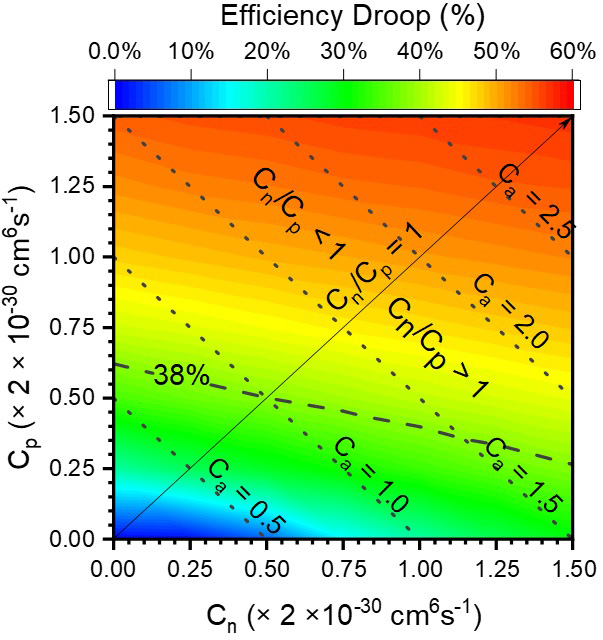29 December 2021

# Effect of Auger electron–hole asymmetry on efficiency droop in InGaN QW LEDs

Indium gallium nitride (InGaN)-based blue light-emitting diodes (LEDs) are the backbone of the solid-state lighting (SSL), but their luminous efficiency peaks under low current densities (<35A/cm2) and rolls off under high current injection levels (efficiency droop), requiring a design tradeoff between light output power, efficiency and cost.

It is widely accepted that Auger recombination is the main cause for the experimentally observed large (~50%) efficiency droop in III–nitride LEDs. Yet there is no clear understanding of the origin of the magnitude of Auger recombination in this material system.

For instance, measured Auger coefficients are obtained by assuming that the electron (n) and hole (p) densities are identical, i.e. n = p. The ambipolar Auger coefficient (Ca) – defined by equality between electron and hole Auger coefficients – is calculated as the sum of the Auger coefficients for the eeh (Cn) and the hhe (Cp) channels, i.e. Ca = Cn + Cp. For piezoelectric materials such as III–nitride LEDs, the carrier symmetry is adversely plagued by the polarization, implying that the Auger electron–hole asymmetry between Cn and Cp (and hence their ratio, Cn/Cp) might play a critical role in understanding the efficiency droop and quantify the Ca. In most experiments and simulations, Cn/Cp is taken as unity (~1), ignoring the effect of Auger electron–hole asymmetry on efficiency droop.

Now, in the paper ‘Effect of Auger Electron–Hole Asymmetry on the Efficiency Droop in InGaN Quantum Well Light-Emitting Diodes’ in the IEEE Journal of Quantum Electronics (https://doi.org/10.1109/JQE.2021.3137822), Y.-C. Tsai, C. Bayram and J.-P. Leburton of University of Illinois at Urbana-Champaign (UIUC) have reported a new Open Boundary Quantum LED Simulator (OBQ-LEDsim) based on variational principles.

By eliminating artificial boundary conditions between quantum wells (QWs) and the classical continuum in LED simulations, OBQ-LEDsim can capture the wavefunction penetration into barriers and other regions. Furthermore, defining the wavefunction continuously enables the incorporation of quantum-confined Stark effects (QCSEs) and excitonic effects into the OBQ-LEDsim.

Using OBQ-LEDsim, Auger recombination is found to already emerge under low current densities because of strong carrier localization. Specifically, the hhe Auger recombination is the primary recombination channel because of the stronger QW localization for holes than for the electrons.

OBQ-LEDsim hence shows that electron–hole asymmetry in Auger recombination is a strong candidate for the origin of the large (~38%) efficiency droop in InGaN-based quantum well LEDs.Picture: Efficiency droop as a function of eeh (Cn) and hhe (Cp) Auger coefficients under the current density of 200A/cm2. Ca, expressed in units of the ambipolar Auger coefficient of 2×10-30 cm6s-1, is plotted as the dotted lines. Multiple solutions for (Cn, Cp) exist for an efficiency droop value. Ignoring the Auger electron–hole asymmetry overestimates the ambipolar Auger coefficient by as much as 62%.

However, increasing Cn/Cp from 0 to ∞ suppresses the hhe Auger recombination, resulting in 25% higher carrier densities. Increased carrier screening fields weaken the QCSE, indicated by a 75% increase in the square wavefunction overlap, and an 8nm blue-shift in the peak emission wavelength. As a result, the efficiency droop is significantly reduced from 48% to 25% as Cn/Cp varies from 0 to ∞. In contrast, if the electron–hole asymmetry is neglected (i.e. Cn/Cp = 1), the ambipolar Auger coefficient Ca is seen to be overestimated by as much as 62%, leading to a misinterpretation in the fundamental limits of InGaN-based LEDs.